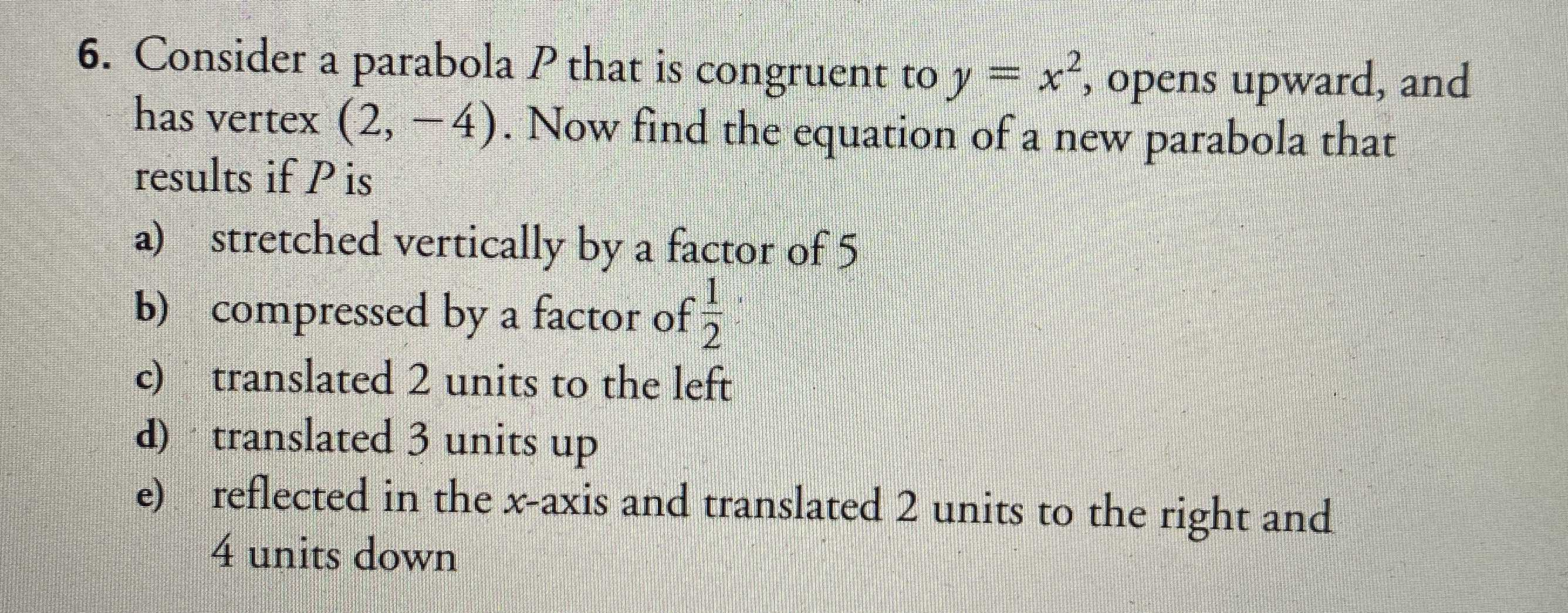### ¿Todavía tienes preguntas de matemáticas?

Pregunte a nuestros tutores expertos
Algebra
Pregunta6. Consider a parabola $$P$$ that is congruent to $$y = x ^ { 2 }$$ , opens upward, and has vertex $$( 2 , - 4 )$$ . Now find the equation of a new parabola that results if $$P$$ is

a) stretched vertically by a factor of $$5$$

b) compressed by a factor of $$\frac { 1 } { 2 }$$

c) translated $$2$$ units to the left

d) translated $$3$$ units up

e) reflected in the $$x$$ -axis and translated $$2$$ units to the right and $$4$$ units down

a) $$y = 5(x - 2)^2 - 4$$

b) $$y = 0.5(x - 2)^2 - 4$$

c) $$y = x^2 - 4$$

d) $$y = (x - 2)^2 - 1$$

e) $$y = - (x - 4)^2 - 8$$

Solución
View full explanation on CameraMath App.